# PSAT Math : How to square a decimal

## Example Questions

### Example Question #2082 : Psat Mathematics

Simplify. Assume all integers are positive real numbers.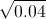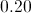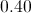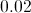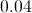Explanation:There are two ways to solve this problem.

First, leave the question in decimal form.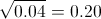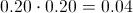Second, put your question in fraction form.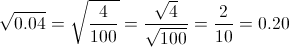### Example Question #1 : How To Square A Decimal

If all real values oflie between 0 and 1, which of the following is always greater than 1?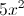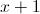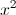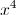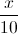Explanation:

Ifis greater than 0, then adding 1 towill make it greater than 1. Taking a number between 0 and 1 to a power results in a smaller number.

### Example Question #2 : How To Square A Decimal

Evaluate:

0.082

0.00064

0.64

0.064

0.0064

0.00064

0.0064

Explanation:

0.08 * 0.08

First square 8:

8 * 8 = 64

Then move the decimal four places to the left:

0.0064